# DAV Class 4 Maths Chapter 4 Worksheet 4 Solutions

The DAV Class 4 Maths Book Solutions and DAV Class 4 Maths Chapter 4 Worksheet 4 Solutions of Division offer comprehensive answers to textbook questions.

## DAV Class 4 Maths Ch 4 WS 4 Solutions

Question 1.
Divide.
(a) 72,895 by 15Q = 4,859
R = 10

(b) 91,027 by 12Q = 7,585
R = 7

(c) 61,526 by 27Q = 2,278
R = 20

(d) 54,325 by 45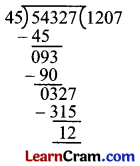Q = 1,207
R = 12

(e) 41,276 by 68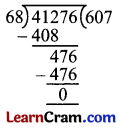Q = 607
R = 0

(f) 91,257 by 54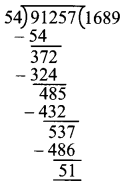Q = 1,689
R = 51Question 2.
Find the quotient and the remainder.
(a) 62,825 ÷ 21Q = 2,991
R = 4

(b) 52,525 ÷ 25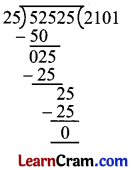Q = 2,101
R = 0

(c) 12,157 ÷ 12Q = 1013
R = 1

(d) 70,012 ÷ 49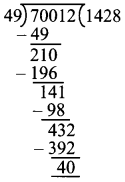Q = 1,428
R = 40

(e) 98,125 ÷ 62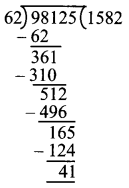Q = 1,582
R = 41

(f) 62,923 ÷ 26Q = 2,420
R = 3

### DAV Class 4 Maths Chapter 4 Worksheet 4 Notes

Division (5-Digit Number by 2 – Digit Number)
Eg: Divide 78, 137 by 25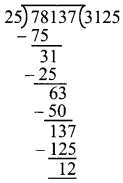Q = 3,125
R = 12
Divisor = 25
Dividend = 78,137

To check:
Dividend = Divisor × Q + R
L.H.S = 78,137
R.H.S = 25 × 3,125 + 12
= 78,125 + 12
= 78,137
∴ L.H.S = R.H.S